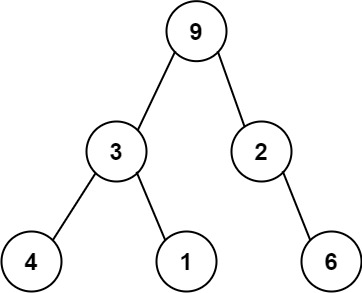# 331. Verify Preorder Serialization of a Binary Tree

Medium
One way to serialize a binary tree is to use preorder traversal. When we encounter a non-null node, we record the node's value. If it is a null node, we record using a sentinel value such as `'#'`.For example, the above binary tree can be serialized to the string `"9,3,4,#,#,1,#,#,2,#,6,#,#"`, where `'#'` represents a null node.
Given a string of comma-separated values `preorder`, return `true` if it is a correct preorder traversal serialization of a binary tree.
It is guaranteed that each comma-separated value in the string must be either an integer or a character `'#'` representing null pointer.
You may assume that the input format is always valid.
• For example, it could never contain two consecutive commas, such as `"1,,3"`.
Note: You are not allowed to reconstruct the tree.
Example 1:
Input: preorder = "9,3,4,#,#,1,#,#,2,#,6,#,#"
Output: true
Example 2:
Input: preorder = "1,#"
Output: false
Example 3:
Input: preorder = "9,#,#,1"
Output: false
Constraints:
• `1 <= preorder.length <= 10^4`
• `preorder` consist of integers in the range `[0, 100]` and `'#'` separated by commas `','`.

### 解題

Runtime: 0 ms, faster than 100%
Memory Usage: 2.2 MB, less than 93.55%
func isValidSerialization(preorder string) bool {
degree := -1
for i:=0; i<len(preorder); i++ {
if preorder[i] == ',' { continue }
if i > 0 && preorder[i - 1] >= '0' && preorder[i - 1] <= '9' { continue }
degree++
if degree > 0 { return false }
if preorder[i] != '#' {
degree -= 2
}
}
return degree == 0
}